# Learn C Language- Tutorial 5: Loop Control Instructions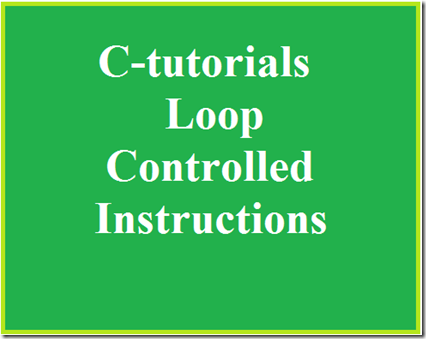So far we have seen the basics of C programming and how to get input from the users and some decision control instructions in the previous tutorials. In this tutorial we will learn about the use of Loop controlled instructions in detail.
Loops are used to execute certain statements repeatedly to until a certain condition is met. This involves repeating some portion of the program in either a specified number of times or until a particular condition is being satisfied.
This repetitive operation is done through a loop control instructions.
There are three methods by way of which we can repeat a part of a program . They are
a) Using a for statement
b) Using a while statement
c) Using a do-while statement

#### While Loop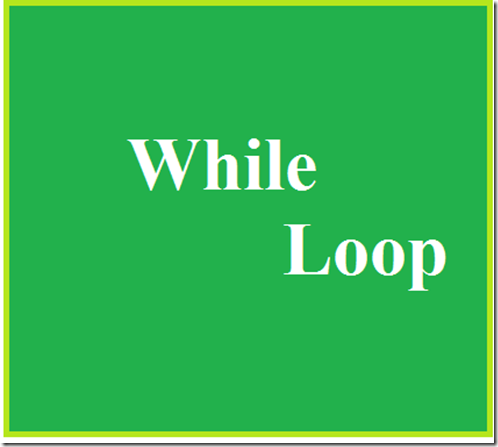It is often the case in programming that you want to do something a fixed number of times. For example getting marks of 5 subjects for 5 students to calculate total marks and generating Ranks among them, getting salaries of n employees and calculating their basic pay, da, etc...
For these purpose we will use this Loop control instructions.
The While loop can be used to solve above situations.
#include<stdio.h>
#include<conio.h>
void main()
{
int p,n,count;
float r,si;
count=1;
while(count<=3)
{
printf(“\n Enter values of p,n,r”);
scanf(“%d%d%f”,&p,&n,&r);
si=p*n*r/100;
printf(“Simple Interest =Rs.%\n f”,si);
count=count+1;
}
return 0;
}
In the above program uses while loop for calculating simple interest for 3 times .
We already know that the first two line of the program is header files , and the next line is the main function from where the actual program starts.
Then the initialization of variables, after that the while loop starts the general form of while loop is that
while(condition)
in this program the condition for while loop is count <=3
it is that the the variable first initialised to the value 1, inside the loop the operation for calculating the simple interest, inside the while condition , the condition is that when the count value incremented from 1 to 2 and 3 the operation must be continued to find the interest till it reaches 3 . Once the count value is reaches 3 the while loops execution stops and the output is displayed .
To increment the count value the line count=count+1
is given. Thus the output is obtained.

#### For LoopIn while loop the initialisation of the variable is outside the loop and it is must to check the condition of the logic , then that variable must be incremented , so that the loop is incremented and the condition is checked until it is satisfied.
It is a very long process, so that the programmers prefer for loop instead of while loop , because this for loop provides initialisation, condition checking and increment in a single line itself.
Syntax for For loop is as follows
for( initialisation ; condition; increment/decrement)
for example
for(i=0;i<=5;i++)
so it is very easy for the programmer to check certain condition.
Let us see an simple program that implements for loop
#include<stdio.h>
#include<conio.h>
void main()
{
int i,n;
clrscr();
printf(“\n Enter the total number :”);
scanf(“%d”,&n);
for(i=1;i<=n;i++)
{
printf(“\n The numbers are %d”,i);
}
getch();
}

Let we code this in an compiler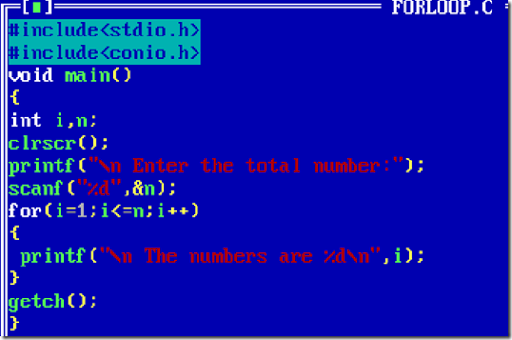Then compile it using Alt+F9 and run it by Ctrl+F9.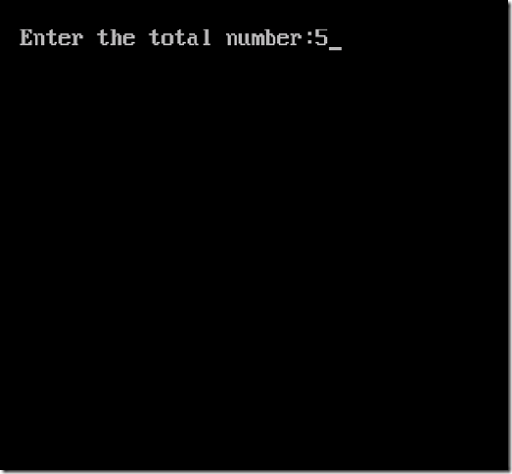provide some numbers then click enter
we get the output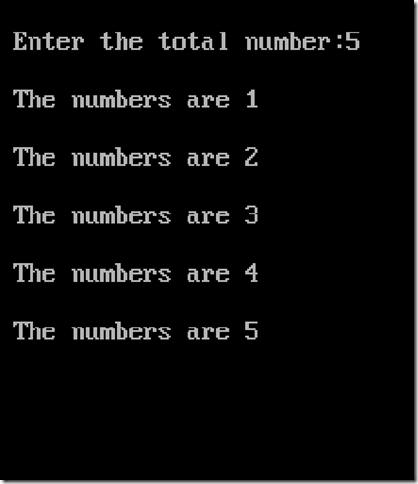Thus we got the output .
Program Explanation:
As we know that the first two lines of the program is including the header files.
Then as usual the void main function, in this program we simply going to get an input number from the user for example and print from 1 to that particular number using the for loop.
For this we need two variables i and n. i is for initialising the values to carry out the for loop and n for getting input from the user.
Then we start the for loop
for(i=1;i<=n;i++)
in this statement i=1 is assigned so that the loop starts from 1 then the i<=n is given so that the loop repeats until the value of i is less and equal to the n , the i++ is incrementing the value of i, so that the loop is executed iteratively.
So , i think you might now understood the importance of the for loop.

#### Do-while Loop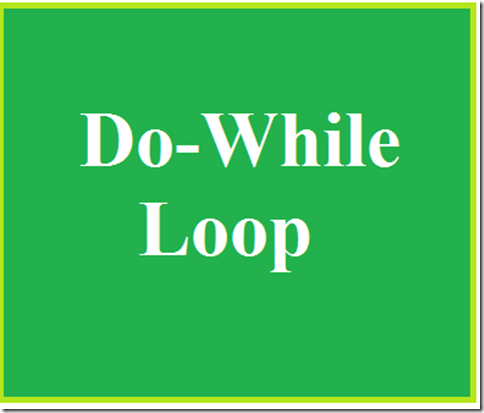There is a minor difference between the working of while and do-while loops. This difference is the place where the condition is tested.
The while  tests the condition before executing any of the statements within while loop.
In do-while tests the condition after having executed the statements within the loop.
The syntax is:
do
{
this;
and this;
and this;
….
}
while(this condition is true);
This means that do-while would execute its statements at least once, even if the condition fails for the first time.
For example to understand the difference between while and do-while consider the following example
#include<stdio.h>
#include<conio.h>
void main()
{
while(4<1)
printf(“Hello world”);
return 0;
}
In this above program the printf statement will not be executed since it does not satisfy the condition .
In case of do-while
#include<stdio.h>
#include<conio.h>
void main()
{
do
{
printf(“\n hello world”);
}while(4<1);
return 0;
}
In the above program the printf statement is executed at east once then only it checks the condition.
Thus the do-while loop can be understood easily.
I think this content is very useful and informative to all the new programmers.